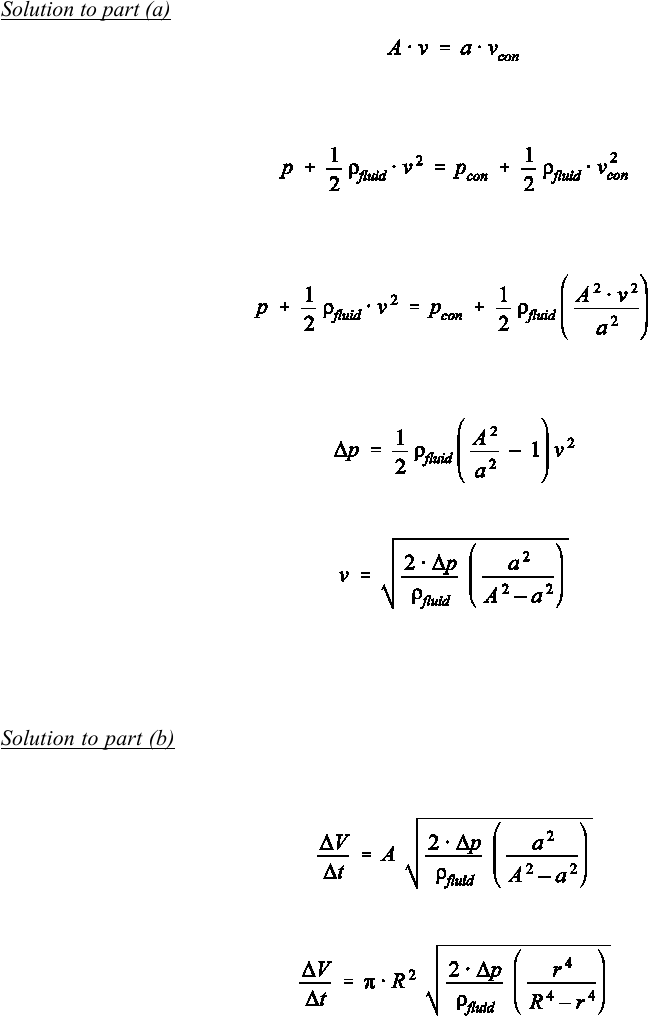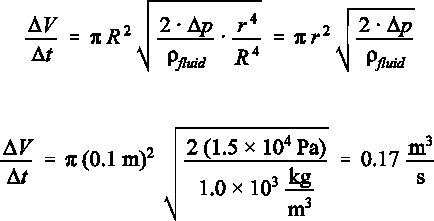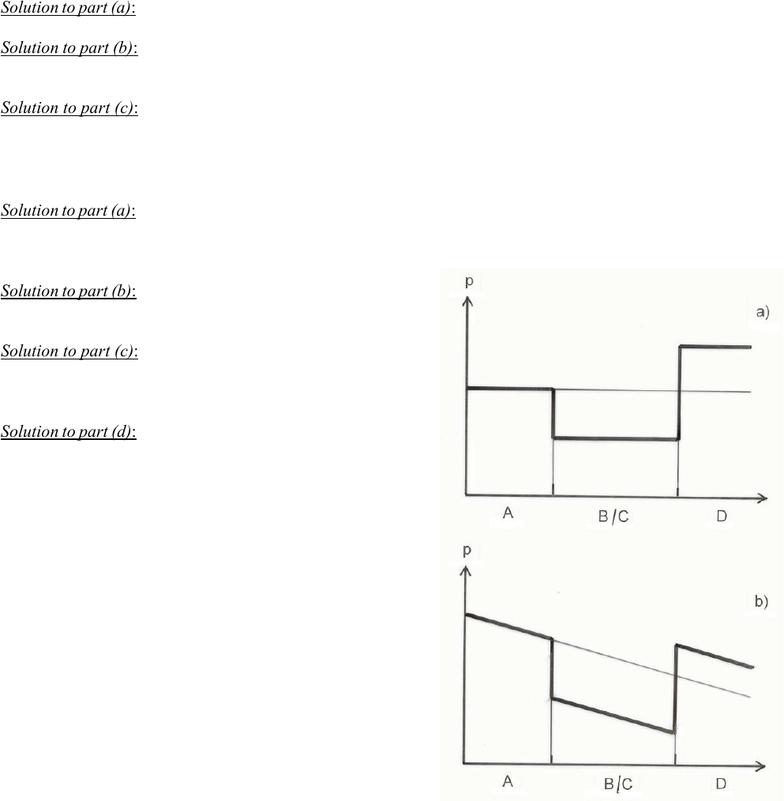# Physics 1028 Chapter Notes - Chapter 13: Viscosity, Newtonian Fluid, International System Of Units

1 views9 pages
School
Western University
Department
Physics
Course
Physics 1028
ProfessorSelection of Even–Numbered Problem Solutions
Chapter 13
P–13.2
Solution to part (a): We use the equation of continuity in the form:
(1)
in which the index con refers to the constricted section of the pipe. The second equation we use is Bernoulli’s
equation in the form:
(2)
We combine both equations by separating the speed of the fluid in the constriction, vcon, in Eq. . This leads
to vcon = A v/a. Then we substitute into Eq. :
(3)
Then we solve for the speed v. We begin by abbreviating the pressure difference as p = ppcon and by
combining the terms which contain v on the right hand side:
(4)
Thus:
(5)
The pressure difference p in Eq. , in turn, is related to the height difference of the liquid in the centre and
left glass tubes by Pascal's law, p = ρliquid A g A h. Replacing p, we get the equation given in the problem
text. Confirm also that the brackets as set in Eq.  are consistent with those in the problem text equation.
Solution to part (b): Note that we do not use the equation from the problem text, but Eq.  to solve this
problem. This equation is rewritten for the volume flow rate V/t using the definition of the volume flow
rate V/t = A v:
(6)
with A = π R² and a = π r²:
(7)
We neglect the r4–term in the denominator of the last bracket in Eq.  since R4 o r4. This simplifies the equa-
tion to:
Unlock document

This preview shows pages 1-3 of the document.
Unlock all 9 pages and 3 million more documents.(8)
Now we substitute the numerical values in this equation:
(9)
Unlock document

This preview shows pages 1-3 of the document.
Unlock all 9 pages and 3 million more documents.P–13.4
Solution to part (a): The fluid density is unchanged since we assume that it is incompressible: ρ = 1.65 g/cm³.
Solution to part (b): We use the equation of continuity and solve for the downstream speed of the fluid. The
result is vdownstream = 11 m/s.
Solution to part (c): We start changing the density and the upstream pressure to SI units. We then use Ber-
noulli’s law and solve for the downstream pressure: p = 2.64 × 104 Pa = 26.4 kPa
P–13.6
Solution to part (a): When the fluid leaves tube A, it enters two equal–sized tubes with a total cross–sectional
area of AB + AC = 2 AB. The speed of the fluid will be the same in each tube because they are indis-
tinguishable. The equation of continuity yields: vA/vB = 2/9.
Solution to part (b): There is no pressure difference because the
diameter doesn’t change along tubes B and C; i.e., p = 0.
Solution to part (c): To quantify this case, we need a value for
η (viscosity coefficient of the fluid), and two geometric pro-
perties of tubes B and C: their inner radius and length.
Solution to part (d): See the attached figure part (a) for the ideal
dynamic fluid and part (b) for a Newtonian fluid.
Unlock document

This preview shows pages 1-3 of the document.
Unlock all 9 pages and 3 million more documents.

## Document Summary

Solution to part (a): we use the equation of continuity in the form: in which the index con refers to the constricted section of the pipe. The second equation we use is bernoulli"s equation in the form: (1) We combine both equations by separating the speed of the fluid in the constriction, vcon, in eq. Then we solve for the speed v. we begin by abbreviating the pressure difference as p = p pcon and by combining the terms which contain v on the right hand side: (3) (2) , in turn, is related to the height difference of the liquid in the centre and left glass tubes by pascal"s law, p = liquid a g a h. Replacing p, we get the equation given in the problem text. Confirm also that the brackets as set in eq.  are consistent with those in the problem text equation.